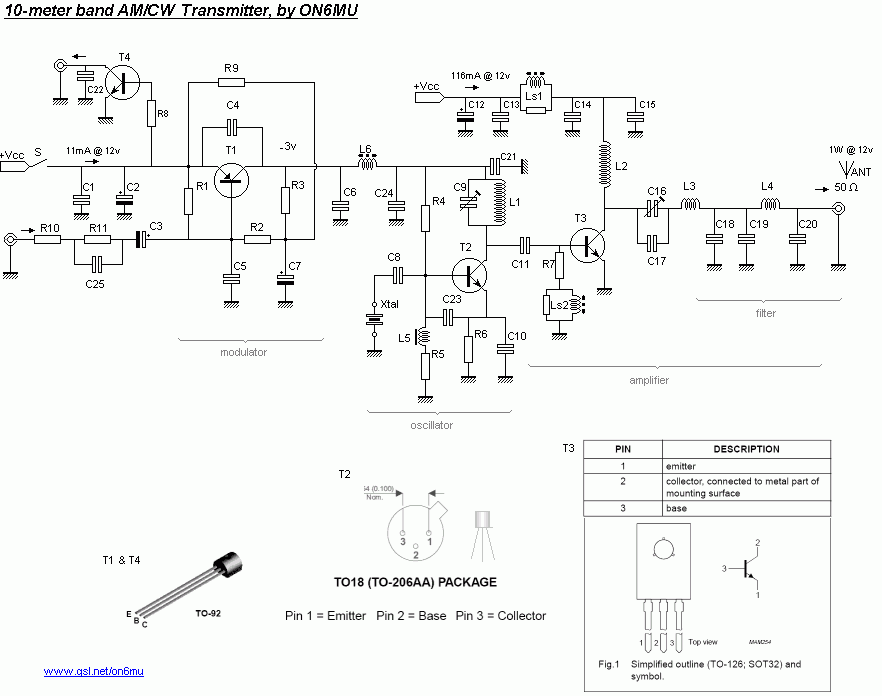# 10米波段1瓦调幅发射机XTAL振荡器，28.xxx XTAL
2KHz的可调频率（如果C8被替换为120PF微调）
LF输入+ / - 100mV（1K频率）

• T1 BC557（调制器）
• T2 BSX20振荡器（2N2219. BC109也可，但少一点功率）
• T3 BD135放大器（带散热片从晶体管隔离）
• T4 2N2222，BC338静音
• C1 = 100nF
• C2 = 47UF/16V（钽）
• C3 = 2.2 uF/50v
• C4 = 33NF（聚酯）
• C5 = 10nF（聚酯）
• C6 = 47nF
• C7 = 4.7uF/50v
• C8 = 10nF
• C9 = 0 ... 22PF（60PF的27MHz）
• C10 = 120PF
• C11 = 56PF
• C12 = 470uF/16v
• C13 = 100nF
• C14 = 47nF
• C15 = 470pF
• C16 = 6 ... 40PF
• C17 = 12pF
• C18 = 120PF
• C19 = 56PF
• C20 = 100pF
• C21 = 470pF
• C22 = 100nF
• C23 = 10pF
• C24 = 33NF
• C25 = 0.47 uF的（聚酯）
• R1 3K9
• R2 3K9
• R3 3K3
• R4 5K6
• R5 1K2
• R6 220
• R7 12
• R8 100K
• R9 4K7
• R10 270
• 390 R11
• LS1，LS2 = 在470 1/2瓦碳膜电阻上，用0.2mm漆包线密绕三层（或2.7UH电感器）
• L1 =0.8毫米绝缘铜线，8.5转并拢的7mm内径
• L2 =0.8毫米绝缘铜线，12转并拢，6毫米内径
• L3 =0.8毫米绝缘铜线，13转并拢的7mm内径
• L4 =0.8毫米绝缘铜线，7轮流并拢的7mm内径
• L5 =100uH电感器
• L6 =100uH电感器
• XTAL基频或倍频为您所需的频率（28 ... 30MHz）
• C4，C5，C6，C25的聚酯薄膜电容器